Welcome to Study Material Solution
Leave Comment

# INTRODUCTION

The world is full of beautiful things. Some of them are natural, whereas some of them are man made. Many of these things are beautiful because they possess symmetry. Symmetry refers to the exact match in shape and size between two halves of an object. That is if we fold a picture in half and both the halves – left half and right half – match exactly then we can say that the picture is symmetrical.

Reflection Symmetry :

If we draw a vertical  line at the middle, the portions on either side of the line are identical. Similarly, in nature we find  many flowers, leaves, etc., that have two identical sides if we draw a line through the middle of them.

Mirror Symmetry :

Reflection symmetry is the symmetry with respect to reflection. If a mirror is placed along the line at the middle, the half part of the figure reflects through the mirror creating the remaining identical half. In other words, the line where the mirror is placed divides the figure into two identical parts they are of the same size and also every specific part on one side of the line will have its reflection exactly at the same distance on the other sides. Thus, it is also known as mirror symmetry or mirror image symmetry.

Line of Symmetry or Axis of Symmetry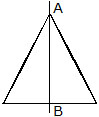The line AB divides the given figures into two identical parts. If a figure is folded along the line AB, one half of the figure will coincide exactly with the other half. In other words, one half is the mirror image of the other half. In such cases we say that the figure is symmetrical and the line which divides the figure into two identical parts is called the line of symmetry or the axis of symmetry.

Two or more lines of symmetry

Some objects and figures have no line of symmetry, a scalene triangle is not symmetrical. We can say that a scalene triangle is asymmetrical.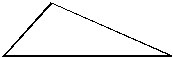(a) Scalene triangle (b) Irregular shape

Some objects and figures have more than one line of symmetry.
Through this isosceles triangle you can draw one line of symmetry.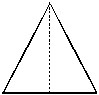A rectangle has two lines of symmetry.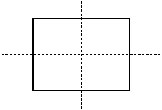If we take an equilateral triangle, we can find that three lines of symmetry can be drawn through the triangle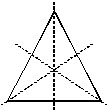A square would have four lines of symmetry,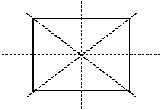A circle has infinite lines of symmetry

OBJECTIVE TYPE

Q.1 Which of the following letters does not have any line symmetry?
(A) H (B) V (C) Z (D) l
Q.2 A rhombus is symmetrical about :
(A) each of its diagonals
(B) the line joining the midpoints of opposite sides
(C) perpendicular bisector of each of the sides
(D) None of these
Q.3 How many lines of symmetry does a rectangle have ?
(A) 1 (B) 2 (C) 4 (D) None
Q.4 Which of the following figures does not have 4 lines of symmetry.
(A)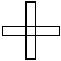(B)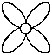(C)(D)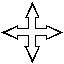Q.5 Which of the following letters has 2 lines of symmetry?
(A) M (B) T (C) X (D) C
Q.6 How many lines of symmetry does a butterfly have ?
(A) 1 (B) 2 (C) 0 (D) 3
Q.7 A triangle which has no lines of symmetry is :
(A) isosceles (B) scalene triangle
(C) equilateral triangle (D) right angled triangle
Q.8 The number of lines of symmetry of the figure  is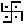(A) 2 (B) 4 (C) 0 (D) 1
Q.9 Which of the following words is made of letters having only horizontal line of symmetry ?
(A) MAT (B) HAT (C) BED (D) MOM
Q.10 Which of the following shapes has more than one line of symmetry ?
(A) Semi-Circle (B) Kite (C) Isosceles trialge (D) Rhombus
Q.11 How many lines of symmetry does a rhombus have ?
(A) 1 (B) 4 (C) 2 (D) 3
Q.12 Alphabet C has :
(A) vertical line of symmetry (B) horizontal line of symmetry
(C) neither vertical nor horizontal (D) no line of symmetry
Q.13 A circle has :
(A) no line of symmetry
(B) infinite lines of symmetry
(C) one line of symmetry
(D) none of these
Q.14 Name the quadrilateral which have only one line of symmetry :
(A) square (B) rhombus (C) kite (D) rectangle
Q.15 Alphabet H has :
(A) vertical line of symmetry (B) horizontal line of symmetry
(C) (A) and (B) both (D) no line of symmetry

SUBJECTIVE TYPE

Q.1 Which of the following have lines of symmetry ? How many lines of symmetry does each have ?
Draw the lines of symmetry in each of the following.
(a)(b)(c)(d)(e)(f)(g)(h)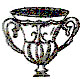(i)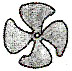Q.2 Draw as many lines of symmetry as possible for each figure.
(a)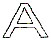(b)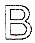(c)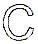(d)(f)(g)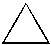(h)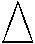(i)

(j)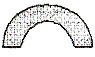(k)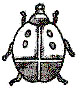(l)(m)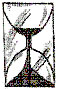(a) Make a list of all the capital alphabets in English which have only one line of symmetry.
(b) Make a list of all the capital alphabets in English which have two lines of symmerty.
(c) Make a list of all the capital alphabets in English which have no line of symmetry.

Q.4 How many axes of symmetry can be drawn through :
(a) the English letter C
(b) an isosceles trapezium
(c) a regular pentagon

(a) no line of symmetry
(b) one line of symmetry
(c) four lines of symmetry

Q.6 Name the lines which are not lines of symmetry in each of the following.

(a)(b)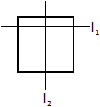(c)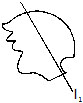(d)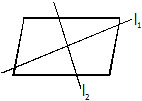(e)(f)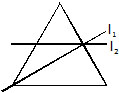(g)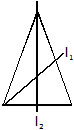(h)(i)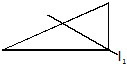OBJECTIVE :

1. C   2. A    3. B   4. C
5. C   6. A    7. B   8. C
9. C  10. D  11.C 12. B
13.B 14. C  15.C

SUBJECTIVE :

1. (a) 1 (b) 2 (c) 6 (d) 1
(e) 2 (f) 0 (g) 6 (h) 1
(i) 0

2. (a) 1 (b) 1 (c) 1 (d) 2
(e) 1 (f) infinite (g) 3 (h) 1
(i) 2 (j) 1 (k) 1 (l) 0
(m) 1

3. (a) A, B, C, D, E, M, T, U, V, W
(b) H, I, X, O
(c) F, G, J, K, L, N, P, Q, R, S, Y, Z

4. (a) 1 (b) 1 (c) 5

5. (a) Any quadrilateral having no equal side
(b) Kite
(c) Square

6. (a) l1 (b) l1 (c) l1 (d) l1, l2
(e) l2 (f) l2 (g) l1 (h) l2
(i) l1

In questions 1 to 17, out of the given four options, only one is correct. Write the correct answer.

1. In the following figures, the figure that is not symmetric with respect to any line is:(A) (i) (B) (ii) (C) (iii) (D) (iv)

2. The number of lines of symmetry in a scalene triangle is
(A) 0 (B) 1 (C) 2 (D) 3

3. The number of lines of symmetry in a circle is
(A) 0 (B) 2 (C) 4 (D) more than 4

4. Which of the following letters does not have the vertical line of symmetry?
(A) M (B) H (C) E (D) V

5. Which of the following letters have both horizontal and vertical lines of symmetry?
(A) X (B) E (C) M (D) K

6. Which of the following letters does not have any line of symmetry?
(A) M (B) S (C) K (D) H

7. Which of the following letters has only one line of symmetry?
(A) H (B) X (C) Z (D) T

8. The instrument to measure an angle is a
(A) Ruler (B) Protractor (C) Divider (D) Compasses

9. The instrument to draw a circle is
(A) Ruler (B) Protractor (C) Divider (D) Compasses

10. Number of set squares in the geometry box is
(A) 0 (B) 1 (C) 2 (D) 3

11. The number of lines of symmetry in a ruler is
(A) 0 (B) 1 (C) 2 (D) 4

12. The number of lines of symmetry in a divider is
(A) 0 (B) 1 (C) 2 (D) 3

13. The number of lines of symmetry in compasses is
(A) 0 (B) 1 (C) 2 (D) 3

14. The number of lines of symmetry in a protractor is
(A) 0 (B) 1 (C) 2 (D) more than 23

15. The number of lines of symmetry in a 45o - 45o - 90o set-square is
(A) 0 (B) 1 (C) 2 (D) 3

16. The number of lines of symmetry in a 30o - 60o - 90o set square is
(A) 0 (B) 1 (C) 2 (D) 3

17. The instrument in the geometry box having the shape of a triangle is called a
(A) Protractor (B) Compasses (C) Divider (D) Set-square

In questions 18 to 42, fill in the blanks to make the statements true.

18. The distance of the image of a point (or an object) from the line of symmetry (mirror) is ________ as that of the point (object) from the line (mirror).

19. The number of lines of symmetry in a picture of Taj Mahal is _______.

20. The number of lines of symmetry in a rectangle and a rhombus are ______ (equal/unequal).

21. The number of lines of symmetry in a rectangle and a square are______(equal/unequal).

22. If a line segment of length 5cm is reflected in a line of symmetry (mirror), then its reflection (image) is a ______ of length _______.

23. If an angle of measure 80o is reflected in a line of symmetry, then thereflection is an ______ of measure _______.

24. The image of a point lying on a line l with respect to the line of symmetry l lies on _______.

25. In Fig. 9.10, if B is the image of the point A with respect to the line l and P is any point lying on
l, then the lengths of line segments PA and PB are _______.26. The number of lines of symmetry in Figure is__________.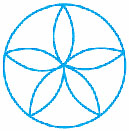27. The common properties in the two set-squares of a geometry box are that they have a __________ angle and they are of the shape of a __________.

28. The digits having only two lines of symmetry are_________ and __________.

29. The digit having only one line of symmetry is __________.

30. The number of digits having no line of symmetry is_________.

31. The number of capital letters of the English alphabets having only vertical line of symmetry is________.

32. The number of capital letters of the English alphabets having only horizontal line of symmetry is________.

33. The number of capital letters of the English alphabets having both horizontal and vertical lines of symmetry is________.

34. The number of capital letters of the English alphabets having no line of symmetry is__________.

35. The line of symmetry of a line segment is the ________ bisector of the line segment.

36. The number of lines of symmetry in a regular hexagon is __________.

37. The number of lines of symmetry in a regular polygon of n sides is_______.

38. A protractor has __________ line/lines of symmetry.

39. A 30o - 60o - 90o set-square has ________ line/lines of symmetry.

40. A 45o - 45o - 90o set-square has _______ line/lines of symmetry.

41. A rhombus is symmetrical about _________.

42. A rectangle is symmetrical about the lines joining the _________ of the opposite sides.

In questions 43 - 61, state whether the statements are true (T) or false (F).

43. A right triangle can have at most one line of symmetry.

44. A kite has two lines of symmetry.

45. A parallelogram has no line of symmetry.

46. If an isosceles triangle has more than one line of symmetry, then it need not be an equilateral triangle.

47. If a rectangle has more than two lines of symmetry, then it must be a square.

48. With ruler and compasses, we can bisect any given line segment.

49. Only one perpendicular bisector can be drawn to a given line segment.

50. Two perpendiculars can be drawn to a given line from a point not lying on it.

51. With a given centre and a given radius, only one circle can be drawn.

52. Using only the two set-squares of the geometry box, an angle of 40o can be drawn.

53. Using only the two set-squares of the geometry box, an angle of 15o can be drawn.

54. If an isosceles triangle has more than one line of symmetry, then it must be an equilateral triangle.

55. A square and a rectangle have the same number of lines of symmetry.

56. A circle has only 16 lines of symmetry.

57. A 45o - 45o - 90o set-square and a protractor have the same number of lines of symmetry.

58. It is possible to draw two bisectors of a given angle.

59. A regular octagon has 10 lines of symmetry.

60. Infinitely many perpendiculars can be drawn to a given ray.

61. Infinitely many perpendicular bisectors can be drawn to a given ray.

62. Is there any line of symmetry in the Fig.  If yes, draw all the lines of symmetry.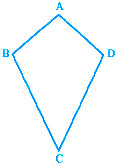63. In Fig. PQRS is a rectangle. State the lines of symmetry of the rectangle.64. Write all the capital letters of the English alphabets which have more than one lines of symmetry.

65. Write the letters of the word ‘MATHEMATICS’ which have no line of symmetry.

66. Write the number of lines of symmetry in each letter of the word ‘SYMMETRY’.

67. Match the following:68. Open your geometry box. There are some drawing tools. Observe them and complete the following table: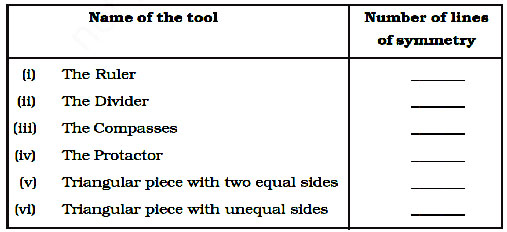1. (b) 2. (a) 3. (d) 4. (c) 5. (b) 6. (b) 7. (d)

8. (b) 9. (b) 10. (c) 11. (c) 12. (b) 13. (a) 14. (b)

15. (b) 16. (a) 17. (d)